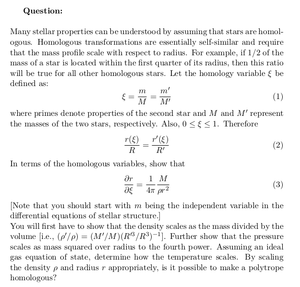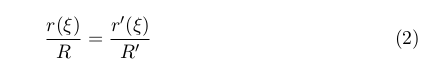Solved Problems in Physics, Physical Chemistry, and Computer Simulation Projects for College and Graduate Students.# Homologous Stars

Regular price
\$12.95
Sale price
\$12.95
Tax included.

Question:
Many stellar properties can be understood by assuming that stars are homologous. Homologous transformations are essentially self-similar and require that the mass profile scale with respect to radius. For example, if 1/2 of the mass of a star is located within the first quarter of its radius, then this ratio will be true for all other homologous stars. Let the homology variable ξ be defined as:where primes denote properties of the second star and M and M' represent the masses of the two stars, respectively. Also, 0 ≤ ξ ≤ 1. ThereforeIn terms of the homologous variables, show that[Note that you should start with m being the independent variable in the differential equations of stellar structure.]

You will first have to show that the density scales as the mass divided by the volume. Further show that the pressure scales as mass squared over radius to the fourth power. Assuming an ideal gas equation of state, determine how the temperature scales. By scaling the density ρ and radius r appropriately, is it possible to make a polytrope homologous?

By purchasing this product, you will get the step by step solution of the above problem in pdf format and the corresponding latex file where you can edit the solution. I am also available to help  you with any possible question you may have.

If you have any question about this solution, please contact to info@solutions4students.com

I'm available to help students with their homework assignments and computer simulation projects.